# iderivative

Derivative of a univariate, vector-valued function using the complex-step approximation.

## Syntax

df = iderivative(f,x0)
df = iderivative(f,x0,h)


## Description

df = iderivative(f,x0) numerically evaluates the derivative of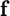with respect to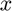at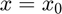using the complex-step approximation with a default step size of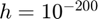.

df = iderivative(f,x0,h) numerically evaluates the derivative ofwith respect toatusing the complex-step approximation with a user-specified step size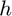.

## Input/Output Parameters

 Variable Symbol Description Format Input f univariate, vector-valued function () 1×1function_handle x0 evaluation point 1×1double h (OPTIONAL) step size 1×1double Output df derivative of with respect to , evaluated at m×1double

## Note

• This function requires 1 evaluation of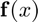.
• If the function is scalar-valued, then.

## Example #1: Derivative of a scalar-valued function.

Approximate the derivative of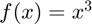at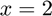using the iderivative function, and compare the result to the true result of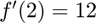.

Approximating the derivative,

f = @(x) x^3;
df = iderivative(f,2)

df =

12



Calculating the error,

error = df-12

error =

0



## Example #2: Derivative of a vector-valued function.

Approximate the derivative of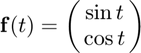at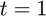using the iderivative function, and compare the result to the true result ofApproximating the derivative,

f = @(t) [sin(t);cos(t)];
df = iderivative(f,1)

df =

0.5403
-0.8415



Calculating the error,

error = df-[cos(1);-sin(1)]

error =

0
0